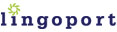# Locale-Sensitive Java Constructor

### `java.lang.Double`

``` Double(double value) Double(String s)```

### Internationalization (I18n) Method Overview

The `Double` class wraps a `double` primitive type in an object. To construct a `Double` object, you pass in either a `double` value or a floating point string.

### I18n Issues

The problem with calling the `Double` constructor with a string parameter is that it is not locale aware. For example, if a string is set to `"2,3"` where a comma is used as the decimal separator, the `Double` constructor will not create the correct `Double` object. This is because it is designed to assume that a period will be used as the decimal separator.

### Suggested Replacement

To ensure that locale is applied when converting between double values and double strings, use the `DecimalFormat` class.

```Double myDouble = new Double(myDecimalString); ```

Use:

``` Double myDouble = null; //Retrieve the runtime user's locale Locale locale = getUserLocale(); //Now call the NumberFormat factory method //and pass the locale object NumberFormat f = NumberFormat.getInstance(locale); //If it is in fact an instance of DecimalFormat, //cast it as such and use it as needed if (f instanceof DecimalFormat) {     try {         myDouble = new Double(((DecimalFormat) f).parse(myDecimalString).doubleValue());     } catch (ParseException e) {} } ```